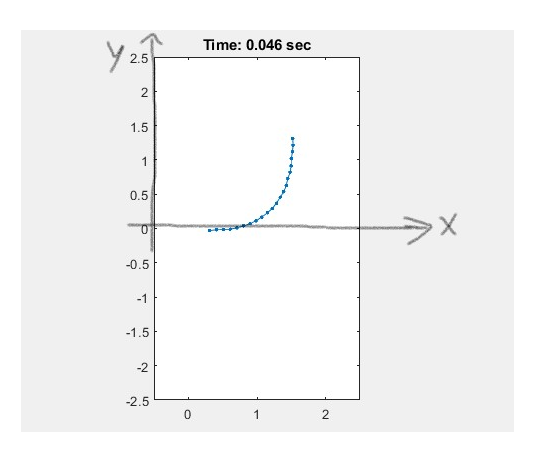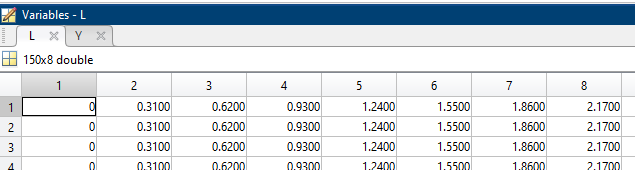grahamq22
Subscriber

Check this post .. it does read a file .. you need to add to this an interpolation functionhttps://forum.ansys.com/discussion/26049/udf-problem-the-expected-wall-controlled-by-an-external-force-do-not-movehttps://forum.ansys.com/discussion/comment/112975#Comment_112975

So I can interpolate it natively? I've only seen examples of people interpolating things like temperature data within UDFs so far. For context, the data is 8 (x,y) ordered pairs varying each time step t, where the x data is equidistant and does not change (only height y changes). The line/wall boundary I want to apply this motion to would have the same length as the range of x, and would lie across the x axis. [frame from animation of line body @ t=0.046]nnSo I would be able to follow steps like in this post (https://www.cfd-online.com/Forums/fluent-udf/176079-fluent-udf-interpolate-material-properties-temp-pressure-dependent-table.html), whereby I read my data with DEFINE_EXECUTE_ON_DEMAND...n... I then write a function to preform a bicubic interpolation, then use DEFINE_PROPERTY to return y (height) continously across the wall's bondary Length/x domain? I assume I would implement DEFINE_GRID_MOTION somewhere here as well.n